Various tips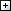Databases
 PictureProgramming languages / configuration
 LinuxVideo
 MKVWindows
 Various tools

# Bash scripting memo

08/03/2023

A memo on useful commands to create a bash script.

## Variables

### Create a variable

a="test"
b=2

To affect the output of a shell command, youcan use \$() :

nb_folder=\$(ls / | wc -l)

### Display une variable

echo \$a

or

echo \${a}

or better, to respect carriage returns if the variable contains some:

echo "\${a}"

### Get the number of characters in a variable

echo \${#a}

-> Display"4" if a="test"

### Extract a substring

You can use the expressions: \${variable:position} ou \${variable:position:length}

echo \${a:2}

-> Display"st" if a="test", because it returns the last X characters.

echo \${a:1:2}

-> Display"es" if a="test" because a substring starts at offset 0.

### Increment a variable

i=\$((i+1))

-> Increase the variable i by 1

### Iterate an array

list_elements=("element 1" "element 2")
for element in ("\${list_elements[@]}")
do
echo "\${i}"
done

-> Displays the 2 elements

### Character substitution

The following expression can be used to directly replace characters, by searching/replacing the first occurrence found : \${variable/search/replace}

var="this is a value"
echo \${var/i/z}

-> Display "thzs is a value"

To perform multiple replacements, add a second slash after the variable name : \${variable//search/replace}

### Change the case of a variable

With substitution, you can convert a variable to uppercase:

\${variable^^}

Also in lowercase:

\${variable,,}

### Predefined variables

There are variables already predefined by the bash interpreter, the most interesting of which are:

NomDescription
\$HOMEUser's home directory
\$PATHValue of environnement variable PATH, which contains search paths
\$\$Process PID
\$PPIDParent process PID
\$IChild process PID
\$PWDCurrent folder (may be different from the script path)
\$SECONDSNumber of seconds since shell start
\$?Return code of the previous command

## Conditions (if ... then ... else)

### One-line condition

if <condition>; then <commands>; fi

or

if <condition>; then ; <commands>; else <commands>; fi

if <condition>
then
<commands>
else
<commands>
fi

or

if <condition>
then
<commands>
elif <condition>
then
<commands>
else
<commands>
fi

## Comparison

### String variables

OperatorDescriptionExample
=Equality
if [ my_variable = "b" ]
!=Difference
if [ my_variable != "b" ]
-zIs empty
if [ -z my_variable ]
-nIs not empty
if [ -n my_variable ]

### Number variables

OperatorDescriptionMathematical equivalentExample
-eqEquality=
if [ var1 -eq var2 ]
-neDifference<>
if [ var1 -ne var2 ]
-gtGreater than>
if [ var1 -gt var2 ]
-geGreater or equal>=
if [ var1 -ge var2 ]
-ltLesser than<
if [ var1 -lt var2 ]
-leLesser or equal<=
if [ var1 -le var2 ]

### Match tests (CASE)

The casecommand allows processing according to a value. Here is an example :

case \${number} in
1)
echo "The number is 1."
;;
2)
echo "The number is 2."
;;
*)
echo "The number is neither 1 nor 2."
;;
esac

It is possible to test several variables, like this:

case "\${number1}-\${number2}" in
1-*)
echo "The 1st number is a 1."
;;
*-2)
echo "The 2nd number is a 2."
;;
*)
echo "The 1st number is not a 1 and the 2nd is not a 2."
;;
esac

### Logical functions

You can use the logical functions NOT, AND, OR with the comparisons. Note that for the logical operators AND and OR, where the conditions are combined, the square brackets must be doubled.

FonctionDescriptionExemple
!NOT: inverts the direction of the test
if [ ! \${a} = \${b} ]
&&AND: combines several mandatory conditions
if [[ \${a} = \${b} && \${b} = \${c} ]]
||OR: combines several optional conditions
if [[ \${a} = \${b} || \${b} = \${c} ]]

## Iteration

### FOR loop

for i in \$(seq 1 5)
do
echo "\${i}"
done

In the above case, we use the seq command, which creates a sequence from 1 to 5.

### WHILE loop

i=1
while [ \$i -le 5 ]
do
echo "\${i}"
i=\$((\$i+1))
done

## File

### Check if a file exists

if [ -f "\${mon_fichier}" ]; then echo "The file exists"; fi

### Check if a file is a symbolic link

if [ -h "\${mon_fichier}" ]; then echo "The file is a link"; fi

### Check if a file has size > 0

if [ -s "\${mon_fichier}" ]; then echo "The file contains data"; fi

### Check if a file is executable

if [ -x "\${mon_fichier}" ]; then echo "The file is executable"; fi

### Compare modification dates

You have to use -nt and -ot between two files to compare the modification dates of two files :

if [ "\${file_1}" -nt "\${file_2}" ]; then echo "\${file_1} exists and is newer than \${file_2}"; fi
if [ "\${file_1}" -ot "\${file_2}" ]; then echo "\${file_1} exists and is older than \${file_2}"; fi

## Directory

### Check if a directory exists

if [ -d "\${mon_repertoire}" ]; then echo "The folder exists"; fi

## Various

### At the start of the Bash script

• Always start a bash script with the line below to define the shell interpreter:
#!/bin/bash
• Check the number of input arguments with this:
if [ \$# -ne 4 ] then
echo "The number of argument is incorrect"
exit 1
fi
• Use .sh or .bash extension
• If you put the following line at the beginning of the script, the bash interpreter will stop the execution of the code as soon as an error is encountered:
set -o errexit
• The interpreter will also stop if you use an undeclared variable if you specify:
set -o nounset
• Use the following line to enable debugging:
set -o xtrace
You can also ensure that if the script is launched with the DEBUG=1 variable, the execution traces of each command are displayed:
# Displays traces if script launched like this:DEBUG=1 ./script.sh
if [[ "\${DEBUG-0}" == "1" ]]; then set -o xtrace; fi

### Check the execution of a program

If you called a program, you can retrieve its return code right after execution and send it back to the caller, like this:

java -jar my_programme.jar
statut=\$? # Get return code from previous line
echo "Return code from java : \${statut}"
exit \${statut}

### Create multi-line variables

If you want to write the value of a variable on several lines, suffix each line with "\", as below:

my_variable="This\
is \
a value \
on several lines"
Dernière modification le 08/03/2023 - Quillevere.net

## Commentaires

No inscription needed if you wish to

## Search in this website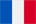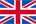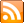RSS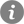Informations